## Example Questions

### Example Question #445 : Algebra

Simplify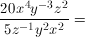None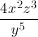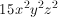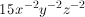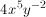Explanation:

Divide the coefficients and subtract the exponents.

### Example Question #446 : Algebra

Which of the following is equal to the expression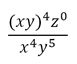, where

xyz ≠ 0?

1/y

z

xyz

z/(xy)

xy

1/y

Explanation:

(xy)4 can be rewritten as x4y4 and z0 = 1 because a number to the zero power equals 1.  After simplifying, you get 1/y.

### Example Question #447 : Algebra

If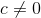, then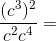Cannot be determined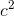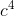Explanation:

Start by simplifying the numerator and denominator separately. In the numerator, (c3)2 is equal to c6. In the denominator, c2 * c4 equals c6 as well. Dividing the numerator by the denominator, c6/c6, gives an answer of 1, because the numerator and the denominator are the equivalent.

### Example Question #448 : Algebra

If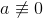, which of the following is equal to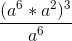?

a

a18

The answer cannot be determined from the above information

a4

a6

a18

Explanation:

The numerator is simplified to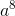(by adding the exponents), then cube the result. a24/a6 can then be simplified to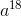.

### Example Question #449 : Algebra

[641/2 + (–8)1/3] * [43/16 – 3171/3169] =

9

30

–30

16

–5

–30

Explanation:

Let's look at the two parts of the multiplication separately. Remember that (–8)1/3 will be negative. Then 641/2 + (–8)1/3 = 8 – 2 = 6.

For the second part, we can cancel some exponents to make this much easier. 43/16 = 43/42 = 4. Similarly, 3171/3169 = 3171–169 = 32 = 9. So 43/16 – 3171/3169 = 4 – 9 = –5.

Together, [641/2 + (–8)1/3] * [43/16 – 3171/3169] = 6 * (–5) = –30.

### Example Question #450 : Algebra

Evaluate: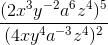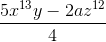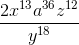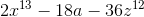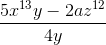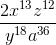Explanation:

Distribute the outside exponents first: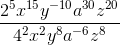Divide the coefficient by subtracting the denominator exponents from the corresponding numerator exponents:### Example Question #1 : Exponential Operations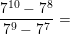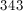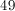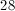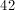Explanation:

The easiest way to solve this is to simplify the fraction as much as possible. We can do this by factoring out the greatest common factor of the numerator and the denominator. In this case, the GCF is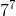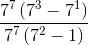Now, we can cancel out thefrom the numerator and denominator and continue simplifying the expression.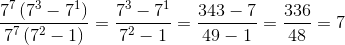Tired of practice problems?

Try live online GRE prep today.